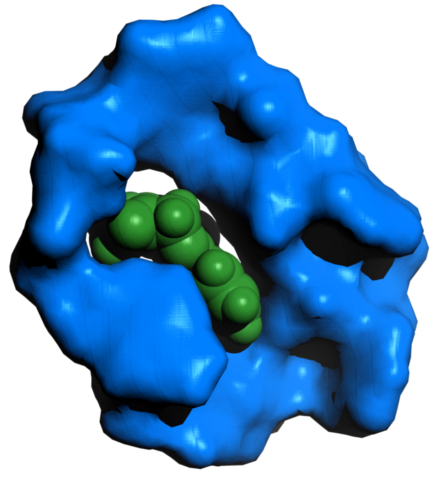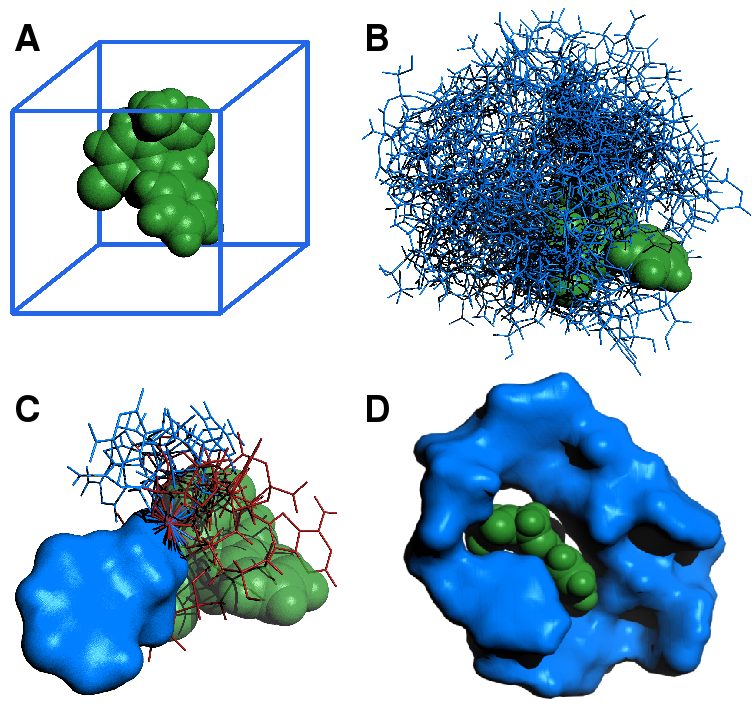# Team:Heidelberg/software/mawsAbstract

Aptamers, originally conceived as a better alternative to antibodies, are now a popular object of research. This research however, is limited by the use of a selection process (SELEX) to generate aptamers. This process needs careful planning and is time consuming with a usual timeframe of multiple weeks. Furthermore, success is heavily dependent on the quality of the nucleic acid library employed in the process. Herein, we construct an entropy-like metric on the space of nowhere zero N-dimensional probability distribution. By using this in an iterative process, we select nucleotides with a probability distribution of maximum distance to the uniform distribution at each step. This yields optimal ligand binding aptamers, without the need of selection. In the field of nucleic acid research, functional nucleic acids have been, and continue to be one of the topics most worked on. Even outside of that field of research, aptamers, ribozymes and DNAzymes are being used to construct very sensitive and specific sensors Rivas2015 Li2013 Zhao2015 for many kinds of molecules, using many kinds of techniques. Yet in all of those approaches, the aptamer constitutes the core of the sensor. Thus, to engineer new sensors for differend kinds of molecules with current experimental methods, one would have to select a nucleic acid library agains those molecules Stoltenburg2007.

Introduction

In the field of nucleic acid research, functional nucleic acids have been, and continue to be one of the topics most worked on. Even outside of that field of research, aptamers, ribozymes and DNAzymes are being used to construct very sensitive and specific sensors Rivas2015 Li2013 Zhao2015 for many kinds of molecules, using many kinds of techniques. Yet in all of those approaches, the aptamer constitutes the core of the sensor. Thus, to engineer new sensors for differend kinds of molecules with current experimental methods, one would have to select a nucleic acid library agains those molecules Stoltenburg2007. That process, known as SELEX (systematic evolution of ligands by exponential enrichment) involves many steps of selection and a well-planned assay, needing time and thought. With time frames of multiple weeks, SELEX is in no way suitable for a fast creation of tests against novel diseases or drugs. Due to this need for a major speedup in the SELEX process, multiple computational approaches were designed. Algorithms have been devised to tackle the problem of nucleic acid library design Luo2010 as well as to simulate the process of SELEX Hu2015 Chushak2009. While the former do not necessarily improve on a good random SELEX pool, the latter needs a significant amount of processing power, requiring four months to set up the selection library on 300 cores Chushak2009.

Software Generated AptamerFigure 1: A diazepam-targeting aptamer binding its ligand.

An aptamer generated by the software MAWS, against the benzodiazepine diazepam, widely known as Valium. The unfolded aptamer was equilibrated with the ligand for 1 ns. The resulting structure was then postprocessed using the PyMol molecular modelling software.

Due to this need for a major speedup in the SELEX process, multiple computational approaches were designed. Algorithms have been devised to tackle the problem of nucleic acid library design Luo2010 as well as to simulate the process of SELEX Hu2015 Chushak2009. While the former do not necessarily improve on a good random SELEX pool, the latter needs a significant amount of processing power, requiring four months to set up the selection library on 300 cores Chushak2009. This obviously makes SELEX the method of choice. In 2011, another approach to the selection of aptamers was demonstrated against thrombin and phosphatidylserine as targets Tseng2011. This method was based on minimizing for each nucleotide in the aptamer its entropy of binding, where entropy was calculated by sampling a grid space of conformations of the aptamer and computing probabilities at this step. Here we expand upon the concept of using entropy to select aptamers by using the maximum entropy expression for probability as an entropy-like distance function to achieve significant improvement in performance. The generated aptamers were experimentally validated and found to be binding their targets.

Algorithm

We first replace the relative entropy $S[P|Q]=-\int{P\cdot{log{\frac{P}{Q}}}d\mathcal{X}}$ by an entropy-like distance using the maximum entropy expression for probability, which is the canonical ensemble $P(\mathcal{X})=\frac{e^{-\beta\cdot{\Delta{G}}}}{Z}$, with $\Delta{G}$ the gibbs free energy of a single conformation of the aptamer ligand complex and $Z=\int{e^{-\beta{\Delta{G}(\mathcal{X})}}d\mathcal{X}}$. That distance we construct in the following:

Given $S[P|Q]=-\int{P\cdot{log{\frac{P}{Q}}}d\mathcal{X}}$, we have $\bar{S}[P|Q]=-\int{P\cdot{ln{\frac{P}{Q}}}d\mathcal{X}}$. From this follows trivially that $S[P|Q]>S[P'|Q]\Rightarrow\bar{S[P|Q]}>\bar{S[P'|Q]}$. Furthermore, we can assume for further considerations that $Q$ is the uniform distribution, and define $S[P]:=S[P|Q]$, where $Q$ is uniform. We then have: $\bar{S[P]}:=-\int{-P\cdot{\beta\Delta{G(\mathcal{X})}}+ln(\frac{Q}{Z})}$ Here, as everywhere hereafter, $\mathcal{X}$ designates a set of variables comprising all the aptamers coordinates. Now, every nucleotide should be scored by $S[P]$ and the distance in quality between two aptamers is given by the metric $g[P,P']=|\bar{S[P']}-\bar{S[P]}|$. Taking this into account, entropy-like fitness functions $\bar{S[P]}$ provide a partial order on the space of all nucleotide sequences $\mathcal{N}$, and $g[P,P']$ provides a metric on $\mathcal{N}$, or more specifically a metric on space of equivalence classes of all nowhere zero probability distributions, with equivalence being defined as: $A=_{S}B \iff S[A]=S[B]: A,B\in{\mathcal{N}}$. We use $g[P,P']$ to choose nucleotide sequences with a certain distance to the minimum $min(S[P])$ of entropies, which is rather intuitively the most specific nucleotide to the target. To use these principles to calculate aptamers to a certain target, we use the following algorithm:

The MAWS AlgorithmFigure 2: MAWS is based upon a simple iteration of sampling and ranking sequences:

The target is loaded into the software. Following this, positions of all possible nucleotides are sampled randomly from a uniform distribution of positions and orientations, with energies being saved at each sample. Following this, probability distribution $P(\mathcal{X})$ for each nucleotide are calculated, using the partition function $Z$. Entropies are then calculated and sequences ranked by entropy. This is iterated, at each step sampling the probability distribution of the aptamer, apprioximated by $P_N(\mathcal{X_N})\approx{\delta(\mathcal{X_{N-1}}-\hat{\mathcal{X_{N-1}}})}\cdot{\frac{e^{-\beta{E(\mathcal{X_N})}}}{Z}}$.

The Steps VisualizedFigure 3: MAWS completes three basic types of tasks, preparation, initial sampling and iterative sampling, visualized here:

(A) The small molecule target diazepam (green) is loaded, and a bounding box (blue) is generated. (B) Positions inside the bounding box, and possible orientations and conformations of each nucleotide (blue) dG, dA, dT or dC are sampled from a uniform distribution. The nucleotide with the lowest entropy is chosen as the starting point for the next step. (C) Given the position of lowest energy of the previous nucleotide (blue surface), possible conformations of the next nucleotide are sampled from a uniform distribution of all torsion angles. The position of minimal energy is chosen by iteration, discarding unfavourable conformations (red), characterized by intersection with the target, or with the aptamer itself. Favourable conformations (blue line) are positively selected. At each step, the sequences with the lowest per-step entropy are selected for the next step. This is iterated. (C) The finished product of the algorithm for the case of diazepam, folded with the ligand for 1 ns.

1. A bounding box is generated around the target (Fig. 3A). The conformational and positional space inside the bounding box is sampled from a uniform distribution for the four possible nucleotides $C = {dAMP, dGMP, dCMP, dTMP}$ (Fig. 3B). At each sample, positions and energies of the system are saved.
2. Optionally, the bounding box is split along one principal direction. The variance $Var(\Delta{G}_{i})$ is calculated for each half $i$ of the bounding box. The space inside the bounding box is sampled again, with probability $P_{i}=\frac{Var(\Delta{G}_{i})}{\Sigma_{j}Var(\Delta{G}_{j})}$ for a sample to come from half $i$ of the bounding box. This can be iterated until sufficient variance reduction is reached. At the last step, save positions and energies at each sample.
3. For each nucleotide $n \in C$, where $n$ is the nucleotide at the 3' end of the aptamer, the partition function $Z$ is calculated as $Z=V\cdot\Sigma_{I}p_{I}\cdot{\Sigma_{J}\frac{e^{-\beta{\Delta{G_{J}}}}}{N_{J}}} : J\in{Samples},I\in{Strata}$, where $V$ is the volume of the sample space

For each nucleotide $n \in C$, the probability distribution is computed as $P_{J}=\frac{e^{-\beta{\Delta{G_{J}}}}}{Z}$

For each nucleotide $n \in C$, the entropy-like fitness function is computed as $S[P]=-V\cdot\Sigma_{I}p_{I}\cdot\Sigma_{J}(-P_{J}\cdot{\beta\Delta{G_{J}}}+ln(\frac{Q}{Z}))$

4. The nucleotide $n$ with the lowest entropy, and all other satisfying $g[P,P_{best}]\lt{g_{threshold}}$ is accepted for the next step.
5. The next steps are iterated $N$ times, where $N$ is the number of nucleotides the aptamer is to be extended by. For each sequence chosen in the previous step, for each nucleotide $n \in C$:
6. The previous conformation of the aptamer is kept fixed, and $n$ is appended to the sequence. Torsion angles inside that nucleotide are sampled from a uniform distribution (Fig. 3C).
7. For each nucleotide $n \in C$, where $n$ is the nucleotide appended in the previous step, the partition function $Z$ is calculated as $Z=V\cdot{\Sigma_{I}\frac{e^{-\beta{\Delta{G_{I}}}}}{N_{I}}} : I\in{Samples}$, where $V$ is the volume of the sample space.

For each nucleotide $n \in C$, the probability distribution is computed as $P_{I}=\frac{e^{-\beta{\Delta{G_{I}}}}}{Z}$

For each nucleotide $n \in C$, the entropy-like fitness function is computed as $S[P]=-V\cdot{\Sigma_{I}(-P_{I}\cdot{\beta\Delta{G_{I}}}+ln(\frac{Q}{Z}))}$

8. The nucleotide with the lowest entropy, and all other satisfying $g[P,P_{best}]\lt{g_{threshold}}$ are accepted for the next step.

Results

Our implementation of the algorithm, termed MAWS (Making Aptamers Without SELEX), was done in Python using the openMM library and its Python bindings for molecular dynamics simulations as well as the mpmath Python library for arbitrary-precision floating point arithmetics. MAWS, as well as its documentation, is freely available as Open Source on our GitHub repository. Using MAWS, we have generated aptamers for several protein targets, including actin, xylanase, p53, and saCas9, as well as small molecule targets, including kanamycin and ketamine. Each of those aptamers was computed within a day at most (saCas9) and two hours at the least, on a laptop computer with only four cores. The aptamers were then experimentally validated by linking them to an HRP-mimicking DNAzyme, yielding a so-called aptabody. Aptamers against protein targets were tested by a modified Western blot procedure, where the aptabody was used in place of a conventional antibody. Aptamers binding small molecules were assayed in solution. Aptamers designed by MAWS were found to be functional, binding their targets in chemoluminescence based assays (link to stefan and stefan). In silico validation of aptamers showed a tendency for them tightly bind their target in molecular dynamics simulations. Furthermore, MAWS predicted a very tight fit of the aptamer to its ligand.

Discussion

As the principle of minimal relative entropy should be universal, one could envision aptamers comprised of biomolecules other than nucleic acids to be generated by the software. In terms of PNA, XNA or other nucleic acid derivatives it should be possible to incorporate them into the software with minor changes to the parameters. Furthermore, the second and the following steps could be changed to a markov chain monte carlo algorithm, which would forego sampling in regions where the free energy $\Delta{G}$ is very large. One could then switch to sampling interaction energy, as conformations prevented from occurring in nature by the laws of physics could not arise if the markov chain transition probability were to be chosen to be energy gradient dependent, therefore avoiding artifacts. As the algorithm can be executed in a very short time on consumer hardware, it could be beneficial to implement master-worker-style parallelization for this software to be able to harvest more aptamers in a shorter time.